×#### Thank you for registering.

One of our academic counsellors will contact you within 1 working day.

Click to Chat

1800-1023-196

+91-120-4616500

CART 0

• 0

MY CART (5)

Use Coupon: CART20 and get 20% off on all online Study Material

ITEM
DETAILS
MRP
DISCOUNT
FINAL PRICE
Total Price: Rs.

There are no items in this cart.
Continue Shopping• Complete JEE Main/Advanced Course and Test Series
• OFFERED PRICE: Rs. 15,900
• View Details

```Chapter 15: Properties of Triangles Exercise – 15.5

Question: 1

State Pythagoras theorem and its converse.

Solution:

The Pythagoras Theorem: In a right triangle, the square of the hypotenuse is always equal to the sum of the squares of the other two sides.

Converse of the Pythagoras Theorem: If the square of one side of a triangle is equal to the sum of the squares of the other two sides, then the triangle is a right triangle, with the angle opposite to the first side as right angle.

Question: 2

In right ∆ABC, the lengths of the legs are given. Find the length of the hypotenuse

(i) a = 6 cm, b = 8 cm

(ii) a = 8 cm, b = 15 cm

(iii) a = 3 cm, b = 4 cm

(iv) a = 2 cm, b =1.5 cm

Solution:

According to the Pythagoras theorem,

(Hypotenuse)2 = (Base)2 + (Height)2

(i) c2 = a2 + b2

c2 = 62 + 82

c2 = 36 + 64 = 100

c = 10 cm

(ii) c2 = a2 + b2

c2 = 82 + 152

c2 = 64 + 225 = 289

c = 17cm

(iii) c2 = a2 + b2

c2 = 32 + 42

c2 = 9 + 16 = 25

c = 5 cm

(iv) c2 = a2 + b2

c2 = 22 + 1.52

c2 = 4 + 2.25 = 6.25

c = 2.5 cm

Question: 3

The hypotenuse of a triangle is 2.5 cm. If one of the sides is 1.5 cm. find the length of the other side.

Solution:

Let the hypotenuse be ” c ” and the other two sides be ” b ” and ” c”.

Using the Pythagoras theorem, we can say that:

c2 = a2 + b2

2.52 = 1.52 + b2

b2 = 6.25 −2.25 = 4

c = 2 cm

Hence, the length of the other side is 2 cm.

Question: 4

A ladder 3.7 m long is placed against a wall in such a way that the foot of the ladder is 1.2 m away from the wall. Find the height of the wall to which the ladder reaches.Solution:

Let the hypotenuse be h.

Using the Pythagoras theorem, we get:

3.72 = 1.22 + h2

h2 = 13.69 – 1.44 = 12.25

h = 3.5 m

Hence, the height of the wall is 3.5 m.

Question: 5

If the sides of a triangle are 3 cm, 4 cm and 6 cm long, determine whether the triangle is right-angled triangle.

Solution:

In the given triangle, the largest side is 6 cm.

We know that in a right angled triangle, the sum of the squares of the smaller sides should be equal to the square of the largest side.

Therefore,

32 + 42 = 9 + 16 = 25

But,

62 = 36

32 + 42 not equal to 62

Hence, the given triangle is not a right angled triangle.

Question: 6

The sides of certain triangles are given below. Determine which of them are right triangles.

(i) a = 7 cm, b = 24 cm and c= 25 cm

(ii) a = 9 cm, b = 16 cm and c = 18 cm

Solution:

(i) We know that in a right angled triangle, the square of the largest side is equal to the sum of the squares of the smaller sides.

Here, the larger side is c, which is 25 cm.

c2 = 625

We have:

a2+ b2 = 72 + 242 = 49 + 576 = 625 = c2

Thus, the given triangle is a right triangle.

(ii) We know that in a right angled triangle, the square of the largest side is equal to the sum of the squares of the smaller sides.

Here, the larger side is c, which is 18 cm.

c2 = 324

We have:

a2+ b2 = 92+162 = 81 + 256 = 337 not equal to c2

Thus, the given triangle is not a right triangle.

Question: 7

Two poles of heights 6 m and 11 m stand on a plane ground. If the distance between their feet is 12 m.  Find the distance between their tops.

(Hint: Find the hypotenuse of a right triangle having the sides (11 – 6) m = 5 m and 12 m)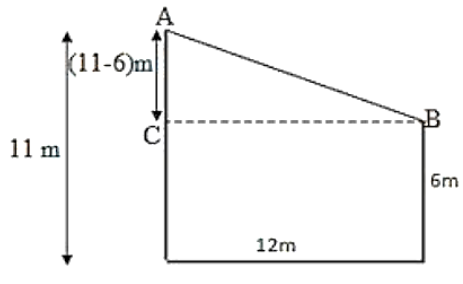Solution:

The distance between the tops of the poles is the distance between points A and B.

We can see from the given figure that points A, B and C form a right triangle, with AB as the hypotenuse.

On using the Pythagoras Theorem in ∆ABC, we get:

(11−6)2 + 122 = AB2

AB2 = 25 + 144

AB2 = 169

AB = 13

Hence, the distance between the tops of the poles is 13 m.

Question: 8

A man goes 15 m due west and then 8 m due north. How far is he from the starting point?Solution:

Let O be the starting point and P be the final point.

By using the Pythagoras theorem, we can find the distance OP.

OP2 = 152 + 82

OP2 = 225 + 64

OP2 = 289

OP = 17

Hence, the required distance is 17 m.

Question: 9

The foot of a ladder is 6 m away from a wall and its top reaches a window 8 m above the ground. If the ladder is shifted in such a way that its foot is 8 m away from the wall, to what height does its top reach?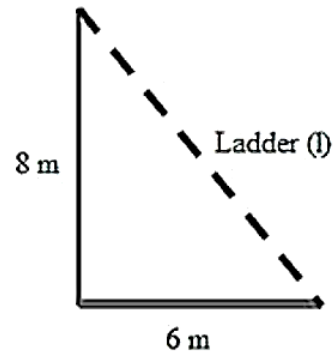Solution:

Given Let the length of the ladder be L m.

By using the Pythagoras theorem, we can find the length of the ladder.

62 + 82 = L2

L2 = 36 + 64 =100

L = 10

Thus, the length of the ladder is 10 m.Let the height of the ladder after it is shifted be H m.

By using the Pythagoras theorem, we can find the height of the ladder after it is shifted.

82 + H2 = 102

H2 = 100 – 64 = 36

H = 6

Thus, the height of the ladder is 6 m.

Question: 10

A ladder 50 dm long when set against the wall of a house just reaches a window at a height of 48 dm. How far is the lower end of the ladder from the base of the wall?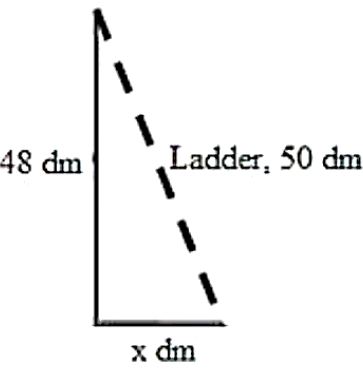Solution:

Let the distance of the lower end of the ladder from the wall be x m.

On using the Pythagoras theorem, we get:

x2 + 482 = 502

x2 = 502 − 482 = 2500 – 2304 = 196

H = 14 dm

Hence, the distance of the lower end of the ladder from the wall is 14 dm.

Question: 11

The two legs of a right triangle are equal and the square of the hypotenuse is 50. Find the length of each leg.

Solution:

Let the length of each leg of the given triangle be x units.

Using the Pythagoras theorem, we get:

x2 + x2 = (Hypotenuse)2

x2 + x2 = 50

2x2 =  50

x2 = 25

x = 5

Hence, we can say that the length of each leg is 5 units.

Question: 12

Verity that the following numbers represent Pythagorean triplet:

(i) 12, 35, 37

(ii) 7, 24, 25

(iii) 27, 36, 45

(iv) 15, 36, 39

Solution:

We will check for a Pythagorean triplet by checking if the square of the largest side is equal to the sum of the squares of the other two sides.

(i) 372 =1369

122 + 352 = 144 + 1225 = 1369

122 + 352 = 372

Yes, they represent a Pythagorean triplet.

(ii) 252 = 625

72 + 242 = 49 + 576 = 625

72 + 242 = 252

Yes, they represent a Pythagorean triplet.

(iii) 452 = 2025

272 + 362 = 729 + 1296 = 2025

272 + 362 = 452

Yes, they represent a Pythagorean triplet.

(iv) 392 = 1521

152 + 362 = 225 + 1296 = 1521

152 + 362 = 392

Yes, they represent a Pythagorean triplet.

Question: 13

In ∆ABC, ∠ABC = 100°, ∠BAC = 35° and BD perpendicular to AC meets side AC in D. If BD = 2 cm, find ∠C, and length DC.Solution:

We know that the sum of all angles of a triangle is 180°

Therefore, for the given ∆ABC, we can say that:

∠ABC + ∠BAC + ∠ACB = 180°

100° + 35° + ∠ACB = 180°

∠ACB = 180° –135°

∠ACB = 45°

∠C = 45°

If we apply the above rule on ∆BCD, we can say that:

∠BCD + ∠BDC + ∠CBD = 180°

45° + 90° + ∠CBD = 180° (∠ACB = ∠BCD and BD parallel to AC)

∠CBD = 180°– 135°

∠CBD = 45°

We know that the sides opposite to equal angles have equal length.

Thus, BD = DC

DC = 2 cm

Question: 14

In a ∆ABC, AD is the altitude from A such that AD = 12 cm. BD = 9 cm and DC = 16 cm. Examine if ∆ABC is right angled at A.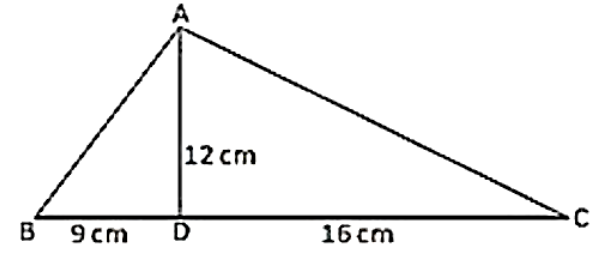Solution:

Using the Pythagoras theorem, we get:

122 + 162 = AC2

AC2 = 144 + 256 = 400

AC = 20 cm

Using the Pythagoras theorem, we get:

122 + 92 = AB2

AB2 = 144 + 81 = 225

AB = 15 cm

In ∆ABC,

BC2 = 252 = 625

AB2 + AC2 = 152 + 202 = 625

AB2 + AC2 = BC2

Because it satisfies the Pythagoras theorem, we can say that ∆ABC is right angled at A.

Question: 15

Draw a triangle ABC, with AC = 4 cm, BC = 3 cm and ∠C = 105°. Measure AB. Is (AC)2 + (BC)2? If not which one of the following is true: (AB)2 > (AC)2 + (BC)2 or (AB)2 < (AC)2 + (BC)2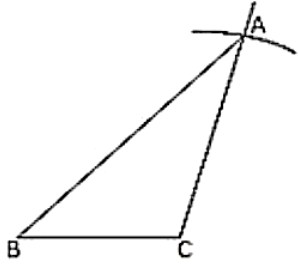Solution:

Draw ∆ABC.

Draw a line BC = 3 cm.

At point C, draw a line at 105° angle with BC.

Take an arc of 4 cm from point C, which will cut the line at point A.

Now, join AB, which will be approximately 5.5 cm.

AC2 + BC2 = 42 + 32 = 9 +16 = 25

AB2 = 5.52 = 30.25

AB2 not equal to AC2+ BC2

Here,

AB2 > AC2 + BC2

Question: 16

Draw a triangle ABC, with AC = 4 cm, BC = 3 cm and ∠C = 80°. Measure AB. Is (AC)2 + (BC)2? If not which one of the following is true: (AB)2 > (AC)2 + (BC)2 or (AB)2 < (AC)2 + (BC)2Solution:

Draw ∆ABC.

Draw a line BC = 3 cm.

At point C, draw a line at 80° angle with BC.

Take an arc of 4 cm from point C, which will cut the line at point A.

Now, join AB; it will be approximately 4.5 cm.

AC2 + BC2 = 42 + 32 = 9 +16 = 25

AB2 = 4.52 = 20.25

AB2 not equal to AC2 + BC2

Here,

AB2 < AC2 + BC2
```### Course Features

• 728 Video Lectures
• Revision Notes
• Previous Year Papers
• Mind Map
• Study Planner
• NCERT Solutions
• Discussion Forum
• Test paper with Video Solution